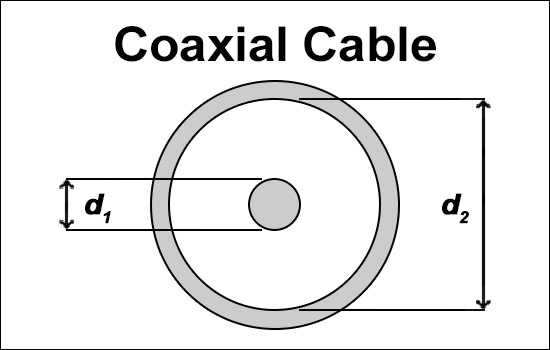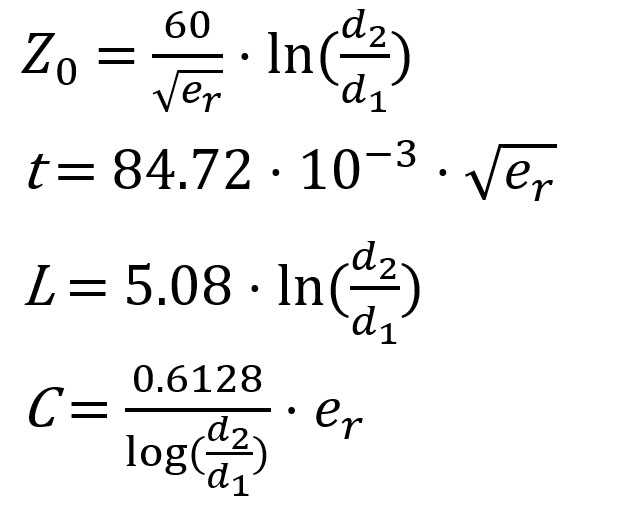# Coaxial Impedance Calculator

This calculator is able to calculate the impedance, delay, inductance, and capacitance of a coaxial cable.

Output values are rounded to the second decimal place.### Input

D1(Inner Cable Diameter):

D2(Sheath Inner Diameter):

Er(Substrate Dielectric):

## EquationsZ = Impedance(Ω), t = Delay(ns/in), L = Inductance(nH/in), C = Capacitance(pF/in)